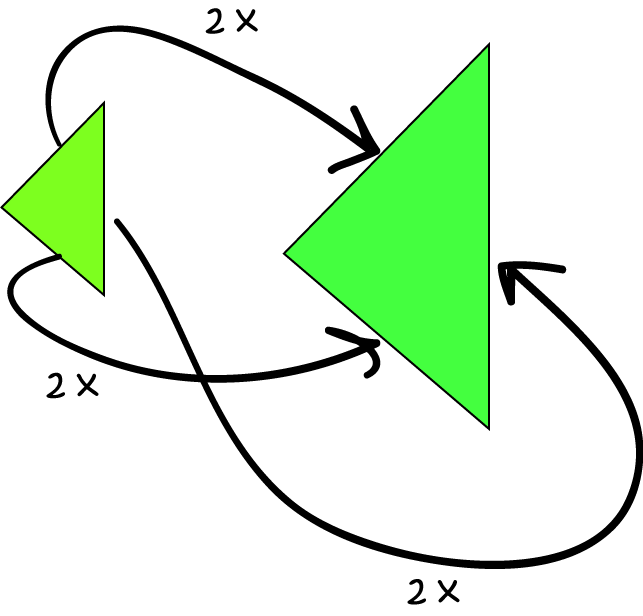# Definition of ProportionalTwo quantities are proportional if the ratio of their sizes is always the same.

For example, if the weight of a sausage is proportional to its length, doubling the weight of the sausage should also double its length, and vice versa.

As another example, the side lengths of the triangles in the picture are proportional to each other. Each side of the bigger triangle has twice the length of the corresponding side of the smaller triangle.

If you want to keep the two triangles proportional to each other and you triple the lengths of the sides of the smaller triangle, you will also have to triple the lengths of the sides of the larger triangle.

### Description

The aim of this dictionary is to provide definitions to common mathematical terms. Students learn a new math skill every week at school, sometimes just before they start a new skill, if they want to look at what a specific term means, this is where this dictionary will become handy and a go-to guide for a student.

### Audience

Year 1 to Year 12 students

### Learning Objectives

Learn common math terms starting with letter P

Author: Subject Coach
You must be logged in as Student to ask a Question.